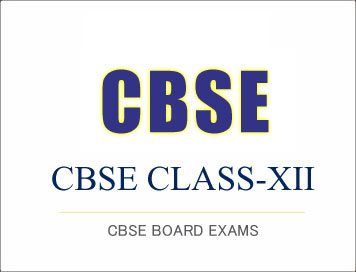Class: XII
Cost Accounting-(781)
Sample Question Paper 2019-20

## SECTION –A

Answer any 10 questions out of the given 12 questions:
1. The document which provides information regarding the progress of each job at each operation is known as:

(b) Job ticket
(c) Job order
(d) None of the above

2. Job No. 21 was in process at the end of the accounting period. The total cost involved in the Job is RS 15,000 of which RS. 5,000 is direct material. Factory overhead is allocated to goods in process at 150% of direct labour cost. Compute the amount of direct labour charged to Job No. 21
(a) RS. 9,000
(b) RS. 4,000
(c) RS. 4,000
(d) RS. 3,000

3. Define Job Costing.

4. Process costing is used in the business where the products are ______________.

5. The cost per unit is the _______________ which is calculated by dividing the total process cost by number of units produced in that particular process.

6. In process costing, each process is debited with material cost, labour cost and ___________ allocated or apportioned to the process.

7. Operating costing is also known as _______________.

8. The transporters may provide services for carrying
(a)Goods
(b)Passengers
(c) Both (a) and (b)
(d) None of the above

9. Interest paid on loan will be debited to ……………..
(a) Cash A/c
(b) Capital A/c
(c) Costing P & L A/c
(d) Financial P & L A/c

10. Do you think that difference in the amount of Depreciation in costing and financial accounting may create difference in Cost Accounting profit from Financial Accounting profit?

11. Payment of donations is Debited to
(b) Manufacturing A/c
(c) Financial P & L A/c
(d) Costing P & L A/c

12. Interest received on investment will be Credited to
(a) Costing P & L A/c
(b) Capital A/c
(c) Financial P & L A/c
(d) Cash A/c